Скачать презентацию Structured products 1 2 3 4 5 6

78ed28ab497ae27818f22af885b7ee1e.ppt

• Количество слайдов: 37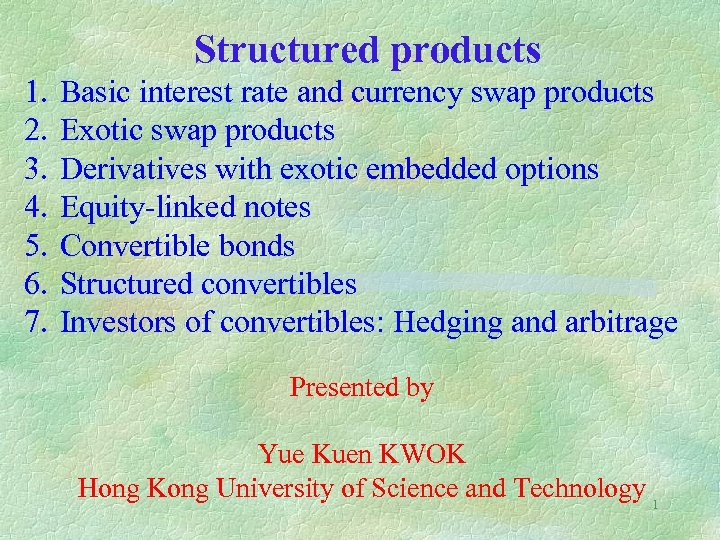Structured products 1. 2. 3. 4. 5. 6. 7. Basic interest rate and currency swap products Exotic swap products Derivatives with exotic embedded options Equity-linked notes Convertible bonds Structured convertibles Investors of convertibles: Hedging and arbitrage Presented by Yue Kuen KWOK Hong Kong University of Science and Technology 1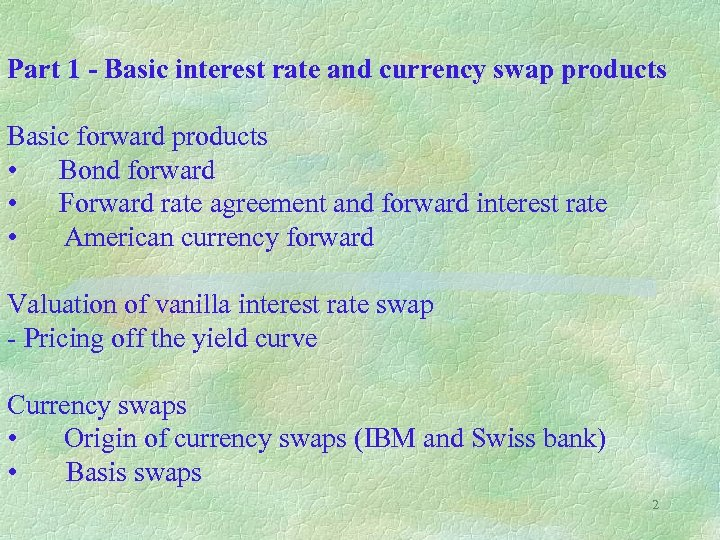Part 1 - Basic interest rate and currency swap products Basic forward products • Bond forward • Forward rate agreement and forward interest rate • American currency forward Valuation of vanilla interest rate swap - Pricing off the yield curve Currency swaps • Origin of currency swaps (IBM and Swiss bank) • Basis swaps 2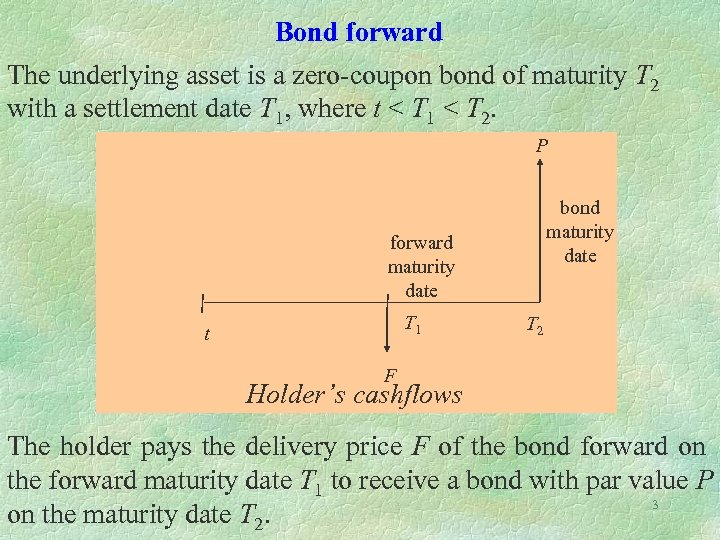Bond forward The underlying asset is a zero-coupon bond of maturity T 2 with a settlement date T 1, where t < T 1 < T 2. P bond maturity date forward maturity date T 1 t T 2 F Holder’s cashflows The holder pays the delivery price F of the bond forward on the forward maturity date T 1 to receive a bond with par value P 3 on the maturity date T 2.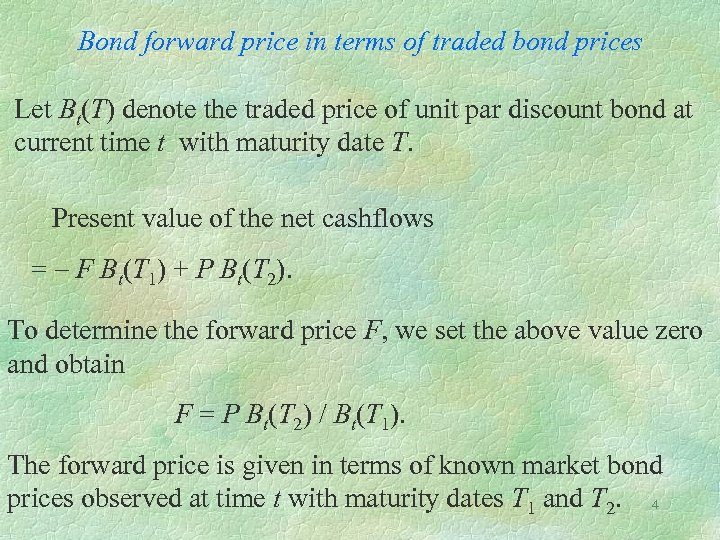Bond forward price in terms of traded bond prices Let Bt(T) denote the traded price of unit par discount bond at current time t with maturity date T. Present value of the net cashflows = - F Bt(T 1) + P Bt(T 2). To determine the forward price F, we set the above value zero and obtain F = P Bt(T 2) / Bt(T 1). The forward price is given in terms of known market bond prices observed at time t with maturity dates T 1 and T 2. 4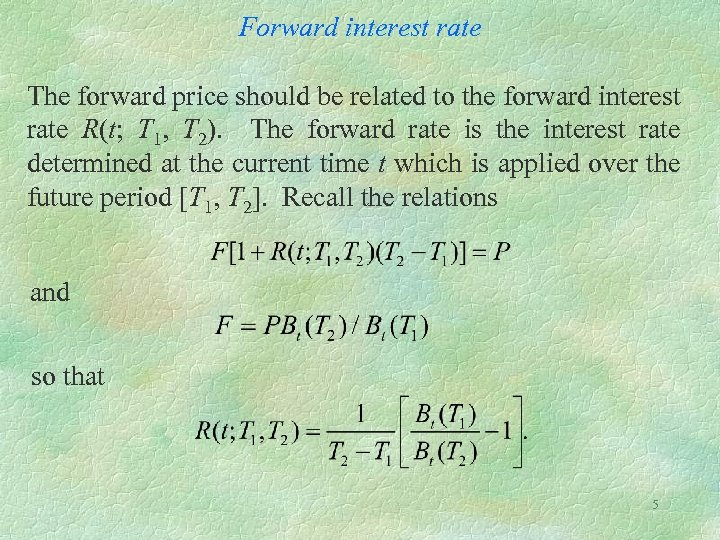Forward interest rate The forward price should be related to the forward interest rate R(t; T 1, T 2). The forward rate is the interest rate determined at the current time t which is applied over the future period [T 1, T 2]. Recall the relations and so that 5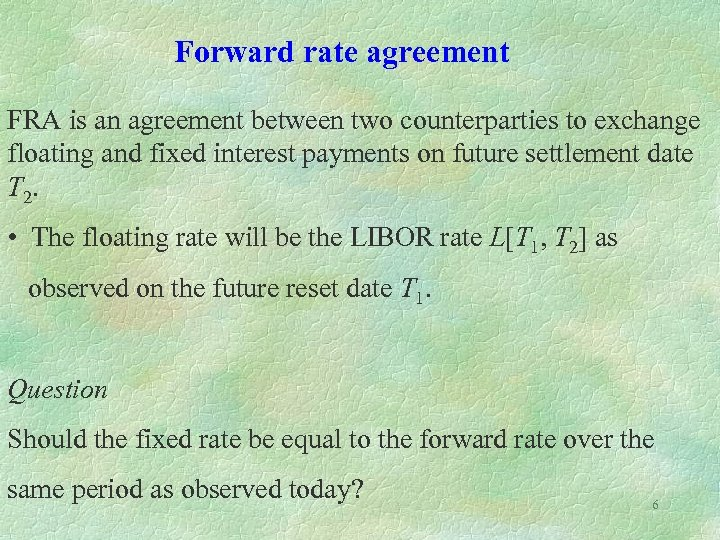Forward rate agreement FRA is an agreement between two counterparties to exchange floating and fixed interest payments on future settlement date T 2. • The floating rate will be the LIBOR rate L[T 1, T 2] as observed on the future reset date T 1. Question Should the fixed rate be equal to the forward rate over the same period as observed today? 6Forward rate agreement L[T 1, T 2] = LIBOR rate observed at future time T 1 for the accrual period [T 1, T 2] K = fixed rate NK(T 2 – T 1) t reset date settlement date T 1 NL(T 1, T 2) (T 2 – T 1) Cashflow of fixed rate receiver 7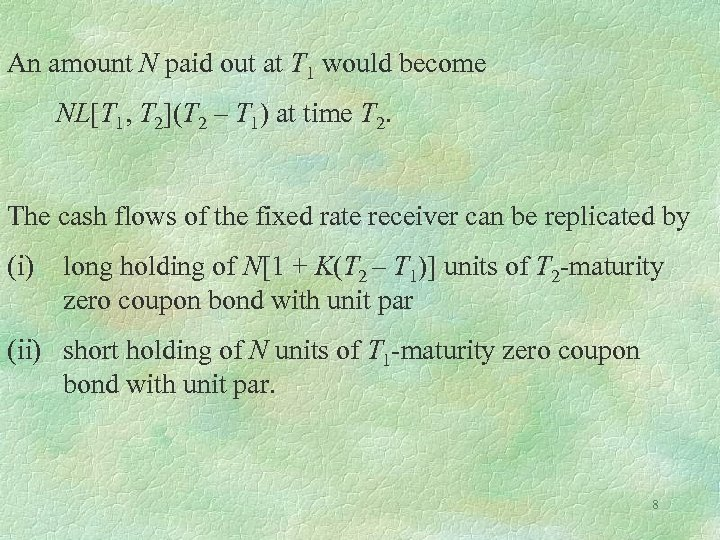An amount N paid out at T 1 would become NL[T 1, T 2](T 2 – T 1) at time T 2. The cash flows of the fixed rate receiver can be replicated by (i) long holding of N[1 + K(T 2 – T 1)] units of T 2 -maturity zero coupon bond with unit par (ii) short holding of N units of T 1 -maturity zero coupon bond with unit par. 8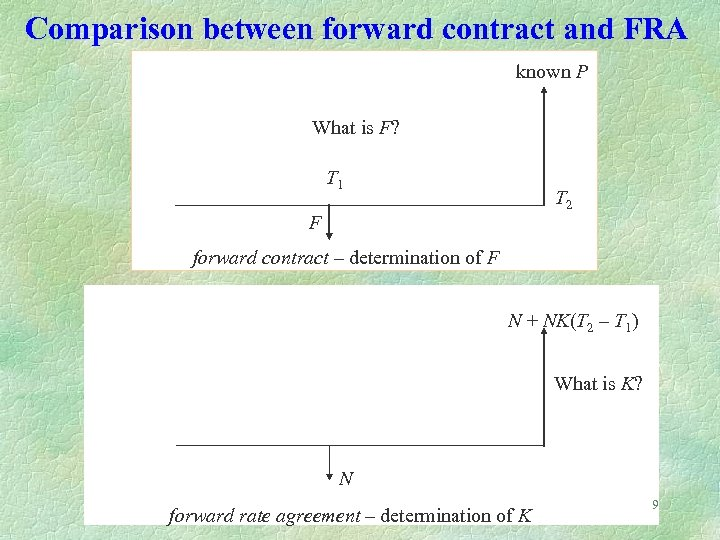Comparison between forward contract and FRA known P What is F? T 1 T 2 F forward contract – determination of F N + NK(T 2 – T 1) What is K? N forward rate agreement – determination of K 9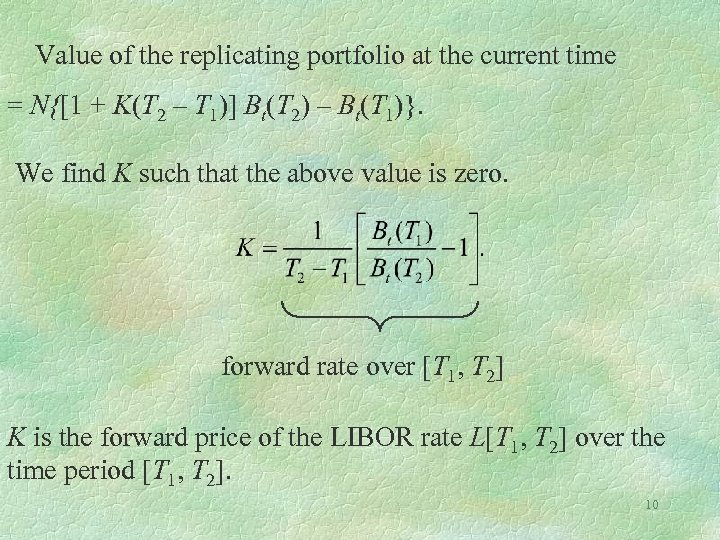Value of the replicating portfolio at the current time = N{[1 + K(T 2 – T 1)] Bt(T 2) – Bt(T 1)}. We find K such that the above value is zero. forward rate over [T 1, T 2] K is the forward price of the LIBOR rate L[T 1, T 2] over the time period [T 1, T 2]. 10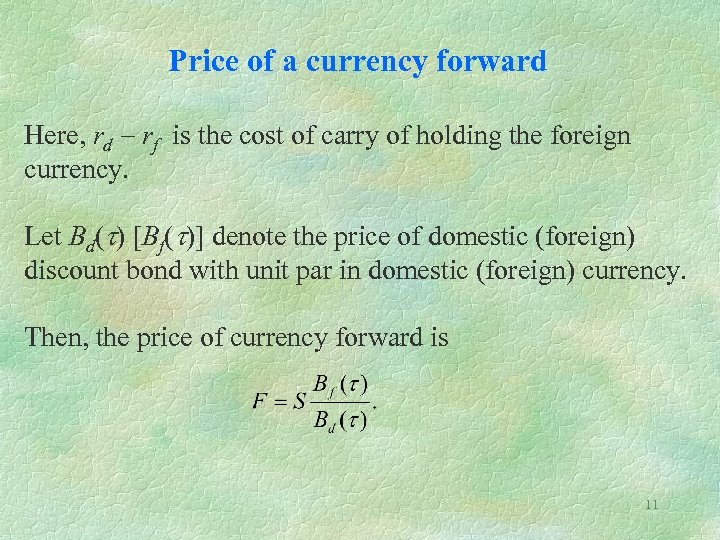Price of a currency forward Here, rd - rf is the cost of carry of holding the foreign currency. Let Bd(t) [Bf(t)] denote the price of domestic (foreign) discount bond with unit par in domestic (foreign) currency. Then, the price of currency forward is 11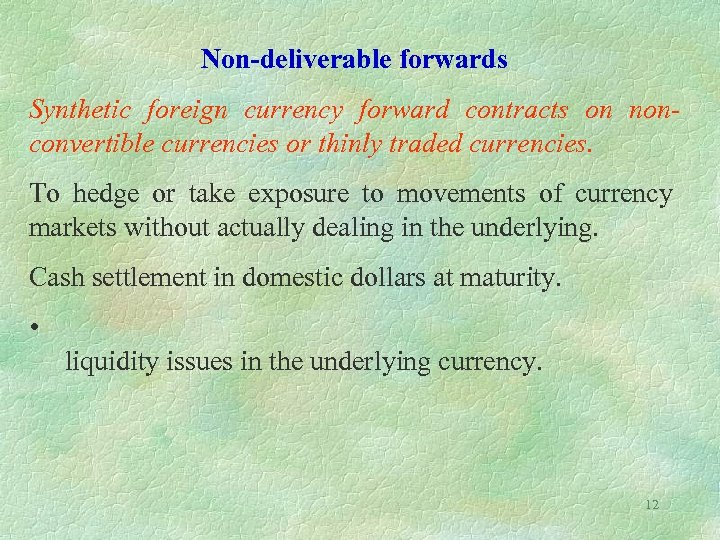Non-deliverable forwards Synthetic foreign currency forward contracts on nonconvertible currencies or thinly traded currencies. To hedge or take exposure to movements of currency markets without actually dealing in the underlying. Cash settlement in domestic dollars at maturity. • liquidity issues in the underlying currency. 12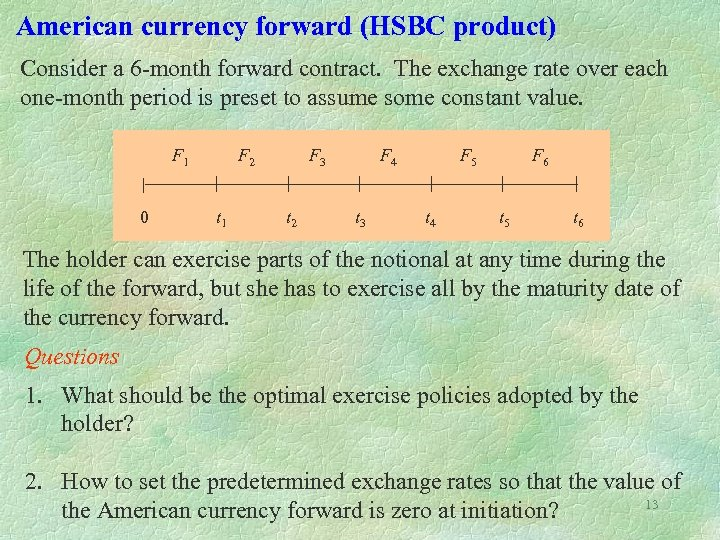American currency forward (HSBC product) Consider a 6 -month forward contract. The exchange rate over each one-month period is preset to assume some constant value. F 1 0 F 2 t 1 F 3 t 2 F 4 t 3 F 5 t 4 F 6 t 5 t 6 The holder can exercise parts of the notional at any time during the life of the forward, but she has to exercise all by the maturity date of the currency forward. Questions 1. What should be the optimal exercise policies adopted by the holder? 2. How to set the predetermined exchange rates so that the value of 13 the American currency forward is zero at initiation?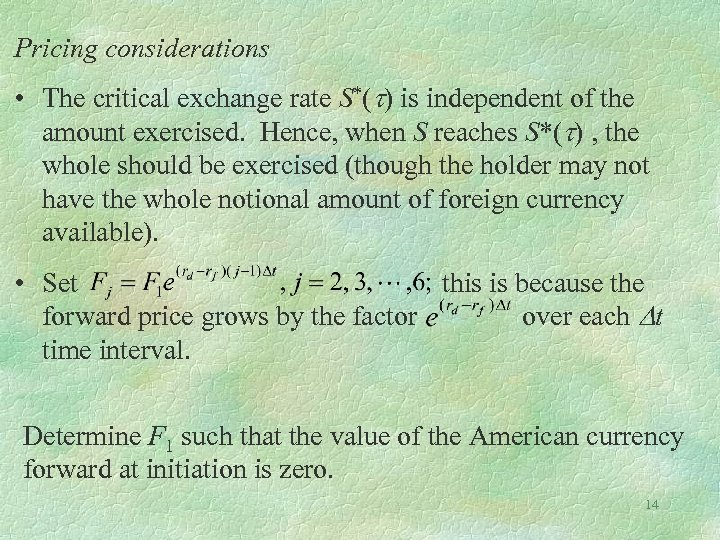Pricing considerations • The critical exchange rate S*(t) is independent of the amount exercised. Hence, when S reaches S*(t) , the whole should be exercised (though the holder may not have the whole notional amount of foreign currency available). • Set this is because the forward price grows by the factor over each Dt time interval. Determine F 1 such that the value of the American currency forward at initiation is zero. 14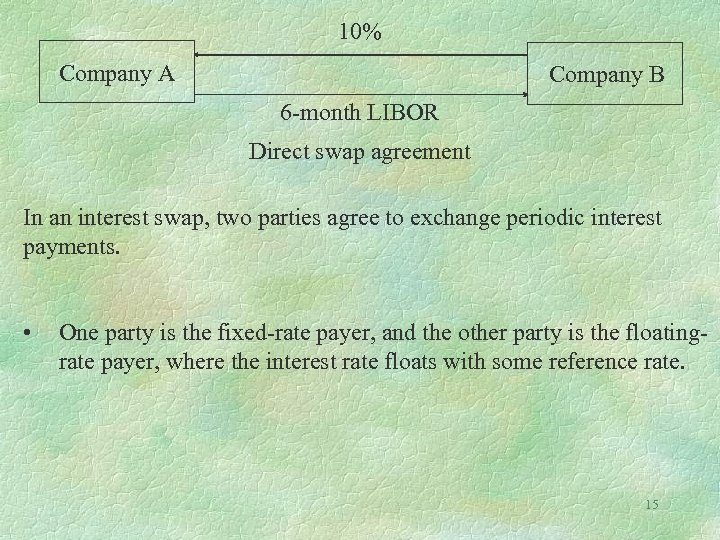10% Company A Company B 6 -month LIBOR Direct swap agreement In an interest swap, two parties agree to exchange periodic interest payments. • One party is the fixed-rate payer, and the other party is the floatingrate payer, where the interest rate floats with some reference rate. 15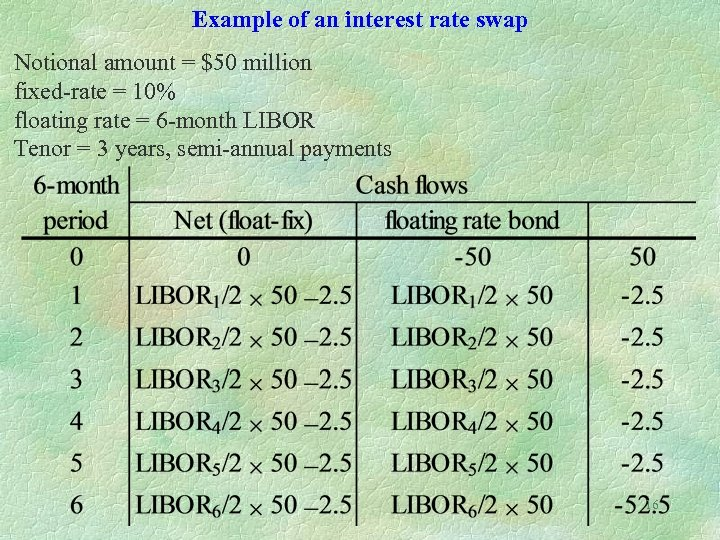Example of an interest rate swap Notional amount = \$50 million fixed-rate = 10% floating rate = 6 -month LIBOR Tenor = 3 years, semi-annual payments 16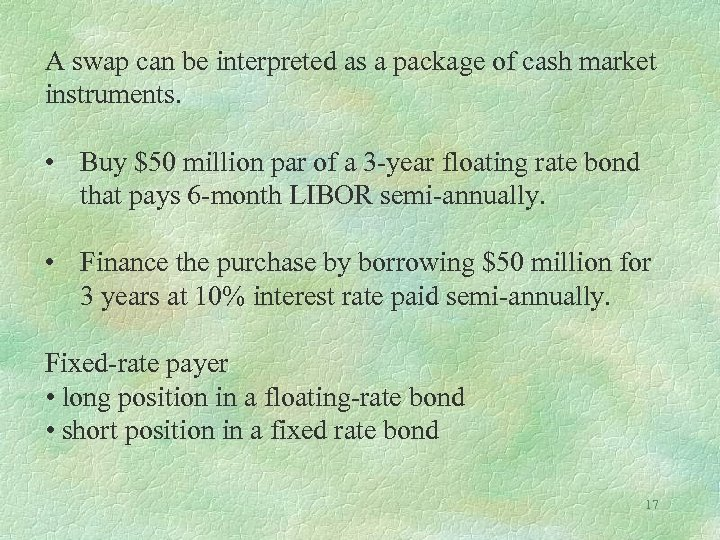A swap can be interpreted as a package of cash market instruments. • Buy \$50 million par of a 3 -year floating rate bond that pays 6 -month LIBOR semi-annually. • Finance the purchase by borrowing \$50 million for 3 years at 10% interest rate paid semi-annually. Fixed-rate payer • long position in a floating-rate bond • short position in a fixed rate bond 17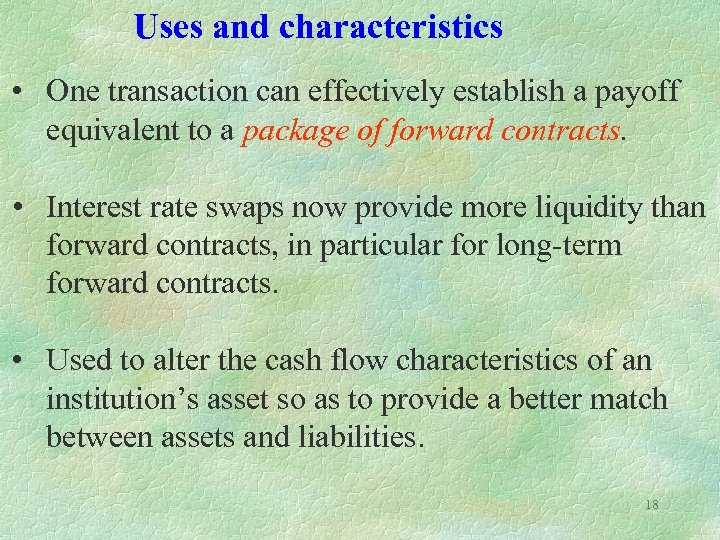Uses and characteristics • One transaction can effectively establish a payoff equivalent to a package of forward contracts. • Interest rate swaps now provide more liquidity than forward contracts, in particular for long-term forward contracts. • Used to alter the cash flow characteristics of an institution’s asset so as to provide a better match between assets and liabilities. 18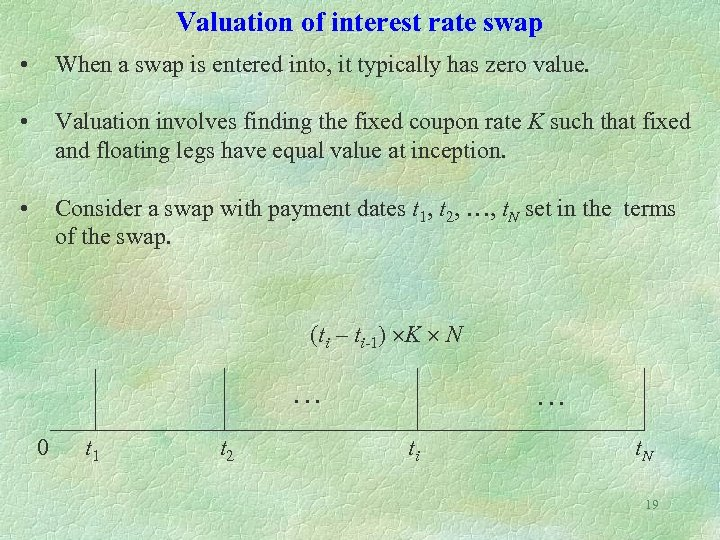Valuation of interest rate swap • When a swap is entered into, it typically has zero value. • Valuation involves finding the fixed coupon rate K such that fixed and floating legs have equal value at inception. • Consider a swap with payment dates t 1, t 2, …, t. N set in the terms of the swap. (ti – ti-1) K N … 0 t 1 t 2 … ti t. N 19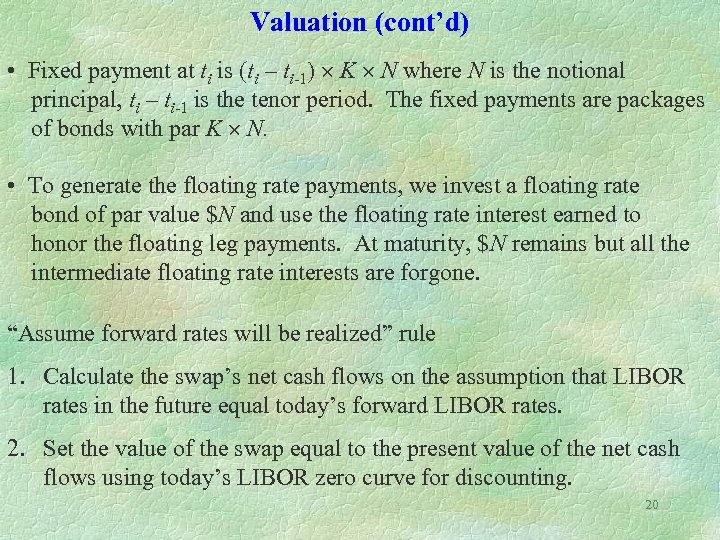Valuation (cont’d) • Fixed payment at ti is (ti – ti-1) K N where N is the notional principal, ti – ti-1 is the tenor period. The fixed payments are packages of bonds with par K N. • To generate the floating rate payments, we invest a floating rate bond of par value \$N and use the floating rate interest earned to honor the floating leg payments. At maturity, \$N remains but all the intermediate floating rate interests are forgone. “Assume forward rates will be realized” rule 1. Calculate the swap’s net cash flows on the assumption that LIBOR rates in the future equal today’s forward LIBOR rates. 2. Set the value of the swap equal to the present value of the net cash flows using today’s LIBOR zero curve for discounting. 20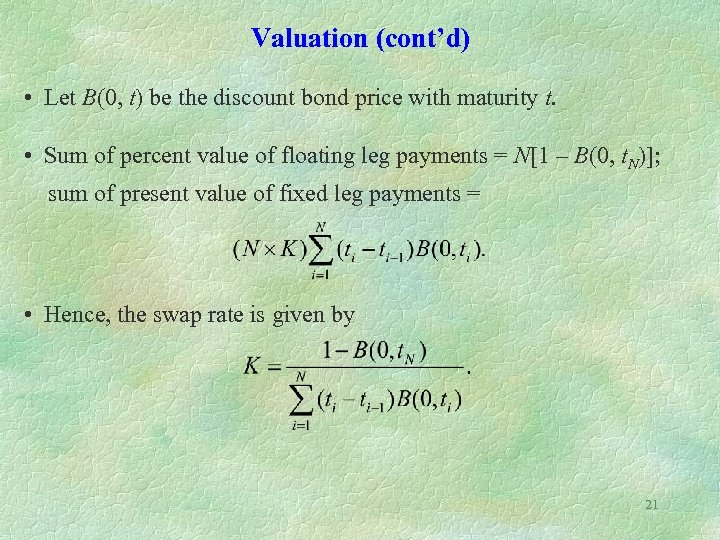Valuation (cont’d) • Let B(0, t) be the discount bond price with maturity t. • Sum of percent value of floating leg payments = N[1 – B(0, t. N)]; sum of present value of fixed leg payments = • Hence, the swap rate is given by 21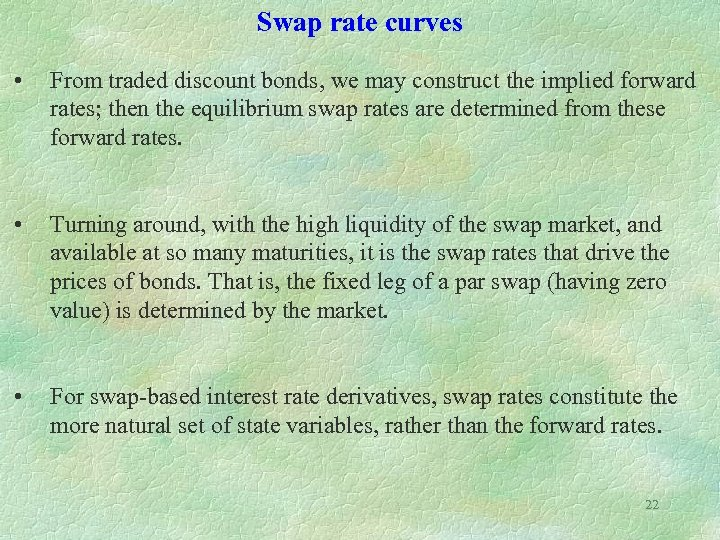Swap rate curves • From traded discount bonds, we may construct the implied forward rates; then the equilibrium swap rates are determined from these forward rates. • Turning around, with the high liquidity of the swap market, and available at so many maturities, it is the swap rates that drive the prices of bonds. That is, the fixed leg of a par swap (having zero value) is determined by the market. • For swap-based interest rate derivatives, swap rates constitute the more natural set of state variables, rather than the forward rates. 22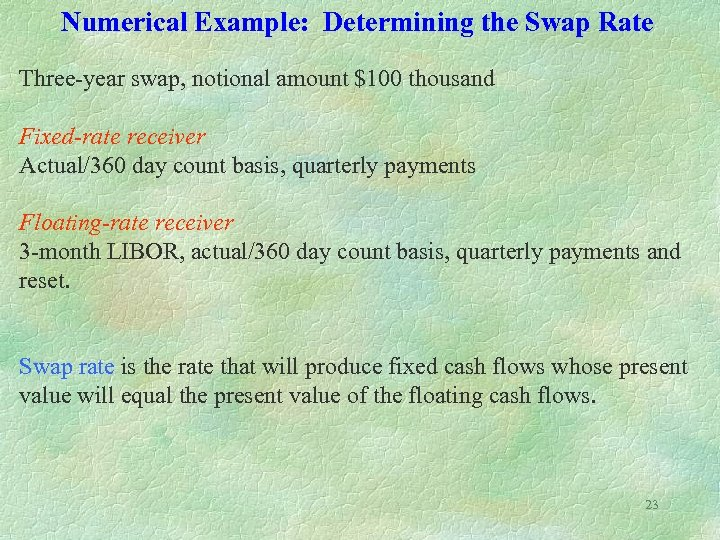Numerical Example: Determining the Swap Rate Three-year swap, notional amount \$100 thousand Fixed-rate receiver Actual/360 day count basis, quarterly payments Floating-rate receiver 3 -month LIBOR, actual/360 day count basis, quarterly payments and reset. Swap rate is the rate that will produce fixed cash flows whose present value will equal the present value of the floating cash flows. 23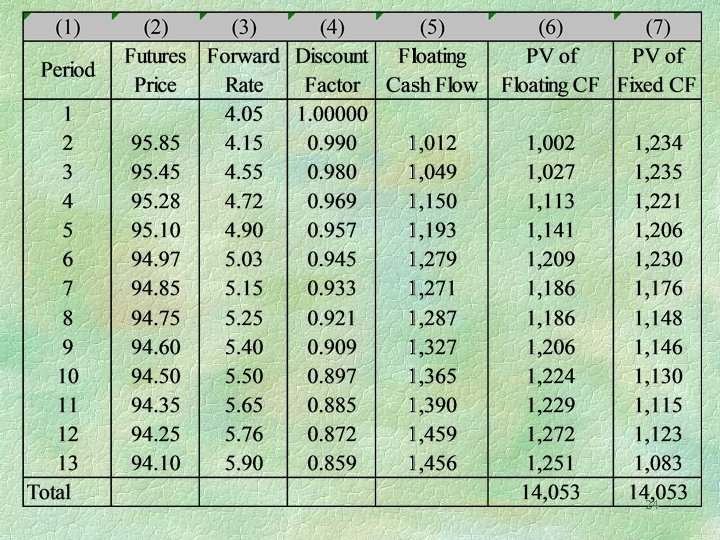24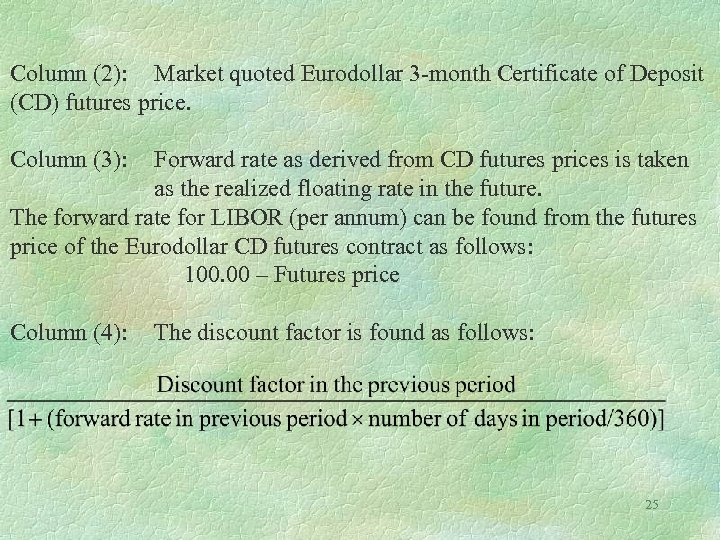Column (2): Market quoted Eurodollar 3 -month Certificate of Deposit (CD) futures price. Column (3): Forward rate as derived from CD futures prices is taken as the realized floating rate in the future. The forward rate for LIBOR (per annum) can be found from the futures price of the Eurodollar CD futures contract as follows: 100. 00 – Futures price Column (4): The discount factor is found as follows: 25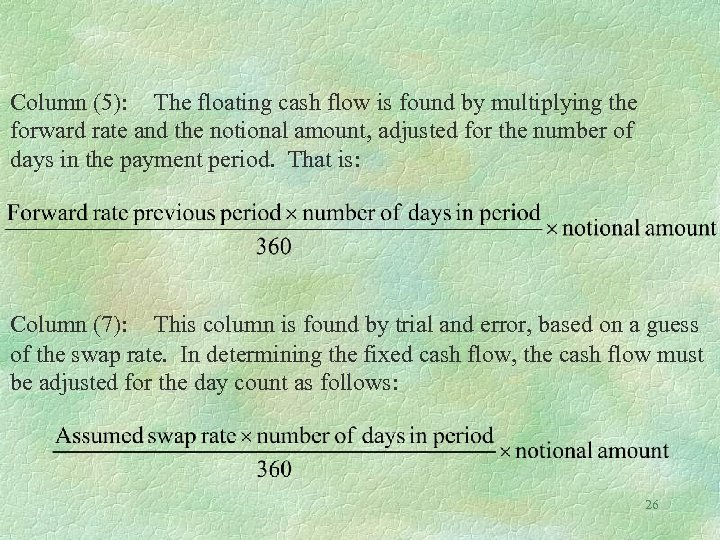Column (5): The floating cash flow is found by multiplying the forward rate and the notional amount, adjusted for the number of days in the payment period. That is: Column (7): This column is found by trial and error, based on a guess of the swap rate. In determining the fixed cash flow, the cash flow must be adjusted for the day count as follows: 26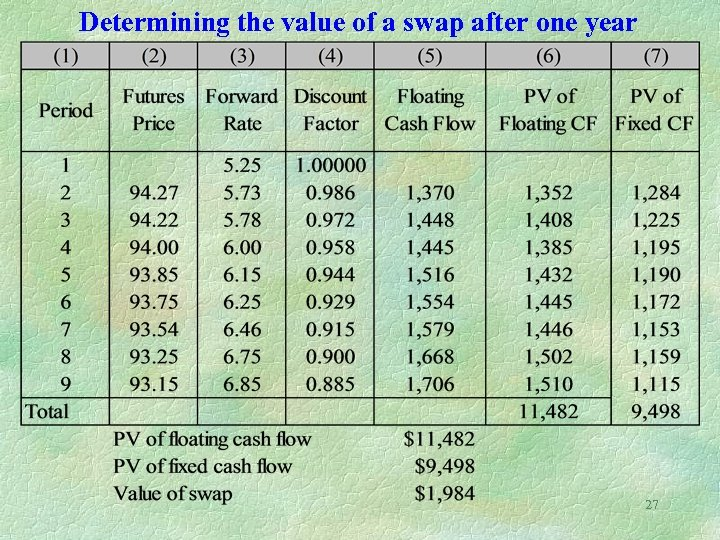Determining the value of a swap after one year 27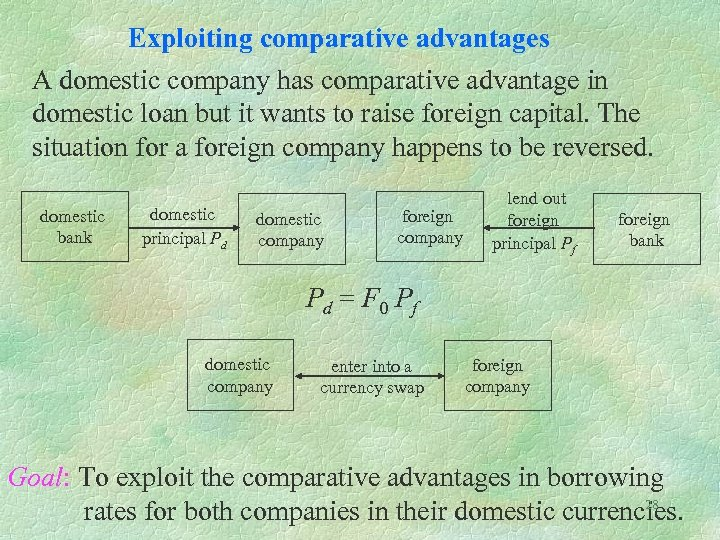Exploiting comparative advantages A domestic company has comparative advantage in domestic loan but it wants to raise foreign capital. The situation for a foreign company happens to be reversed. domestic bank domestic principal Pd domestic company foreign company lend out foreign principal Pf foreign bank Pd = F 0 Pf domestic company enter into a currency swap foreign company Goal: To exploit the comparative advantages in borrowing 28 rates for both companies in their domestic currencies.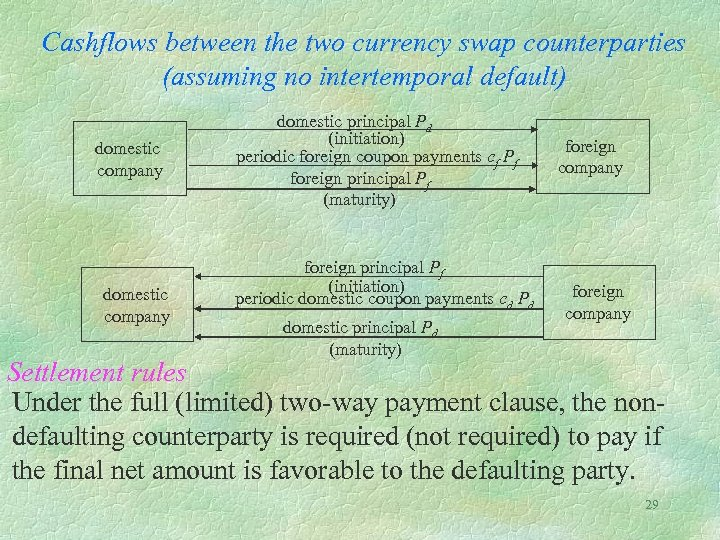Cashflows between the two currency swap counterparties (assuming no intertemporal default) domestic company domestic principal Pd (initiation) periodic foreign coupon payments cf Pf foreign principal Pf (maturity) foreign principal Pf (initiation) periodic domestic coupon payments cd Pd domestic principal Pd (maturity) foreign company Settlement rules Under the full (limited) two-way payment clause, the nondefaulting counterparty is required (not required) to pay if the final net amount is favorable to the defaulting party. 29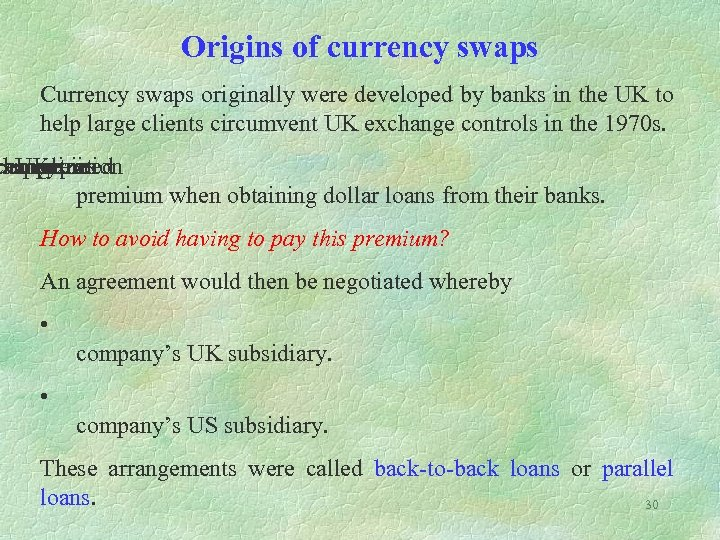Origins of currency swaps Currency swaps originally were developed by banks in the UK to help large clients circumvent UK exchange controls in the 1970 s. changeto companies anpay equalization UK required • were premium when obtaining dollar loans from their banks. How to avoid having to pay this premium? An agreement would then be negotiated whereby • company’s UK subsidiary. • company’s US subsidiary. These arrangements were called back-to-back loans or parallel loans. 30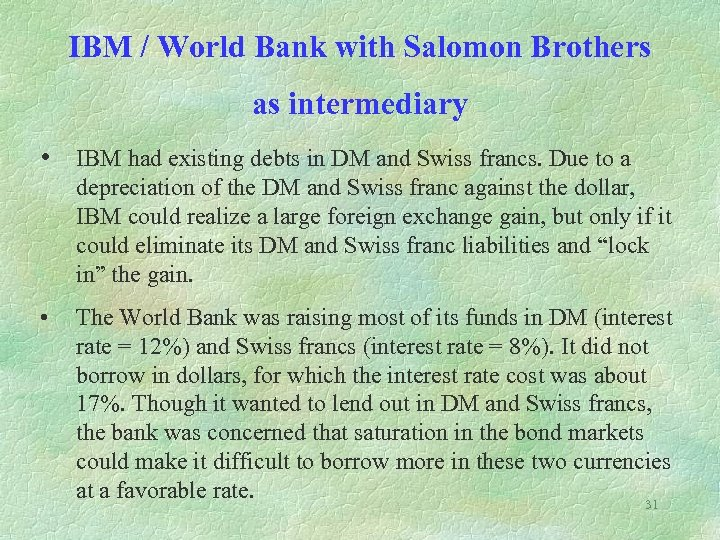IBM / World Bank with Salomon Brothers as intermediary • IBM had existing debts in DM and Swiss francs. Due to a depreciation of the DM and Swiss franc against the dollar, IBM could realize a large foreign exchange gain, but only if it could eliminate its DM and Swiss franc liabilities and “lock in” the gain. • The World Bank was raising most of its funds in DM (interest rate = 12%) and Swiss francs (interest rate = 8%). It did not borrow in dollars, for which the interest rate cost was about 17%. Though it wanted to lend out in DM and Swiss francs, the bank was concerned that saturation in the bond markets could make it difficult to borrow more in these two currencies at a favorable rate. 31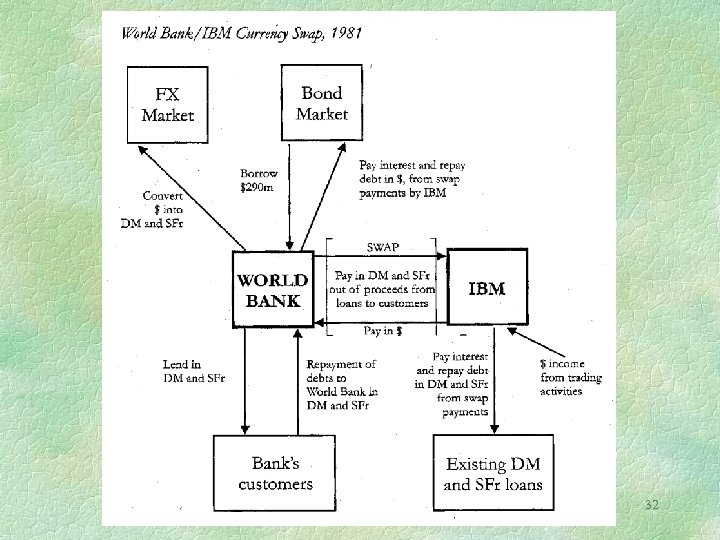32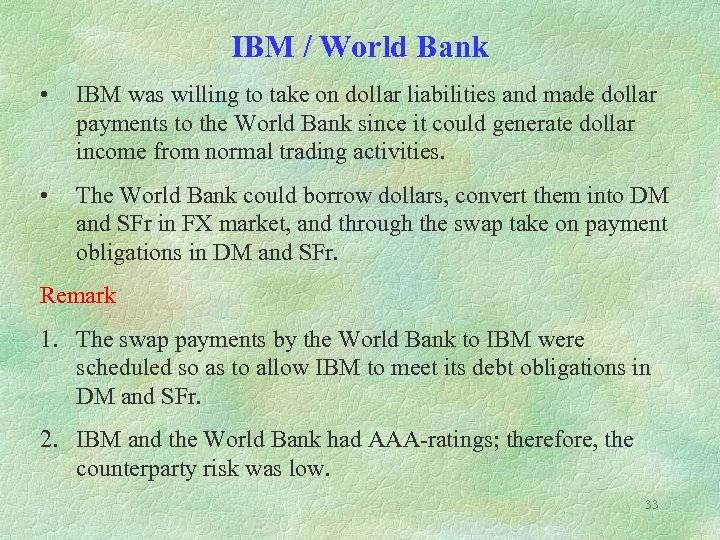IBM / World Bank • IBM was willing to take on dollar liabilities and made dollar payments to the World Bank since it could generate dollar income from normal trading activities. • The World Bank could borrow dollars, convert them into DM and SFr in FX market, and through the swap take on payment obligations in DM and SFr. Remark 1. The swap payments by the World Bank to IBM were scheduled so as to allow IBM to meet its debt obligations in DM and SFr. 2. IBM and the World Bank had AAA-ratings; therefore, the counterparty risk was low. 33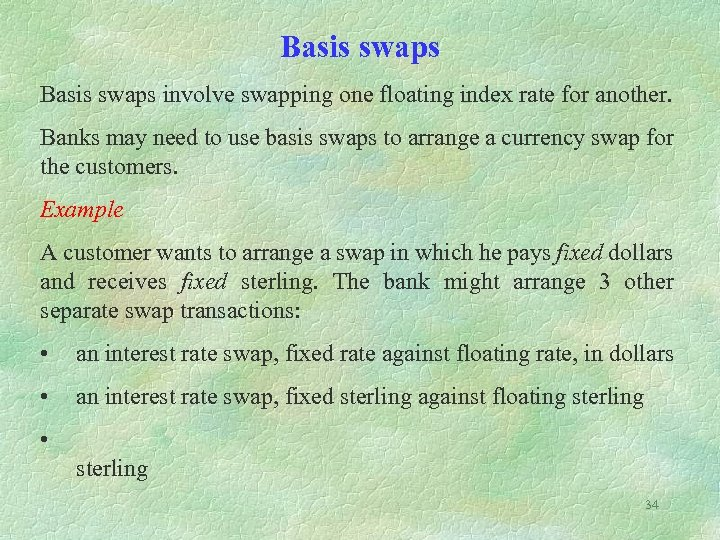Basis swaps involve swapping one floating index rate for another. Banks may need to use basis swaps to arrange a currency swap for the customers. Example A customer wants to arrange a swap in which he pays fixed dollars and receives fixed sterling. The bank might arrange 3 other separate swap transactions: • an interest rate swap, fixed rate against floating rate, in dollars • an interest rate swap, fixed sterling against floating sterling • sterling 34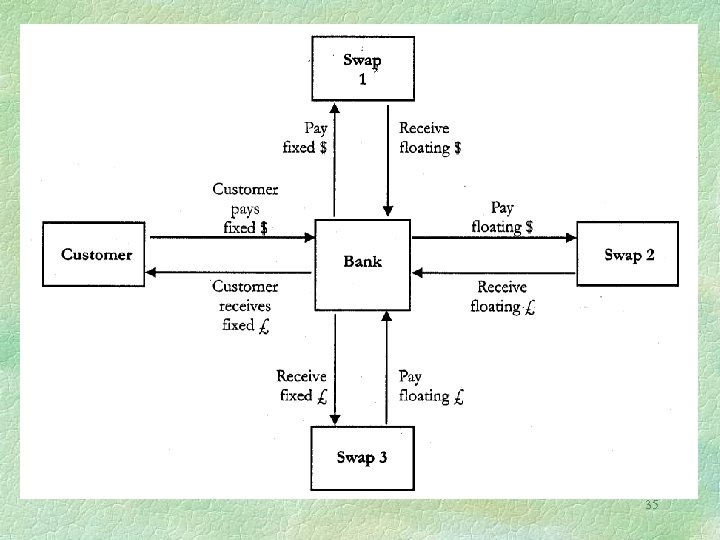35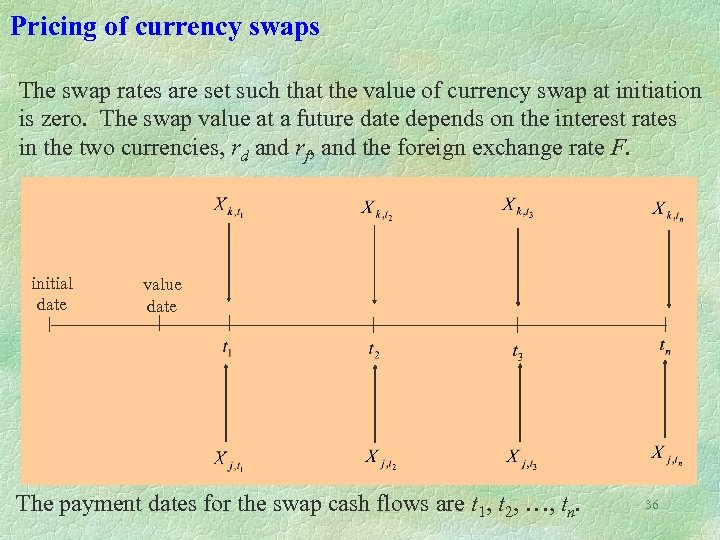Pricing of currency swaps The swap rates are set such that the value of currency swap at initiation is zero. The swap value at a future date depends on the interest rates in the two currencies, rd and rf, and the foreign exchange rate F. initial date value date The payment dates for the swap cash flows are t 1, t 2, …, tn. 36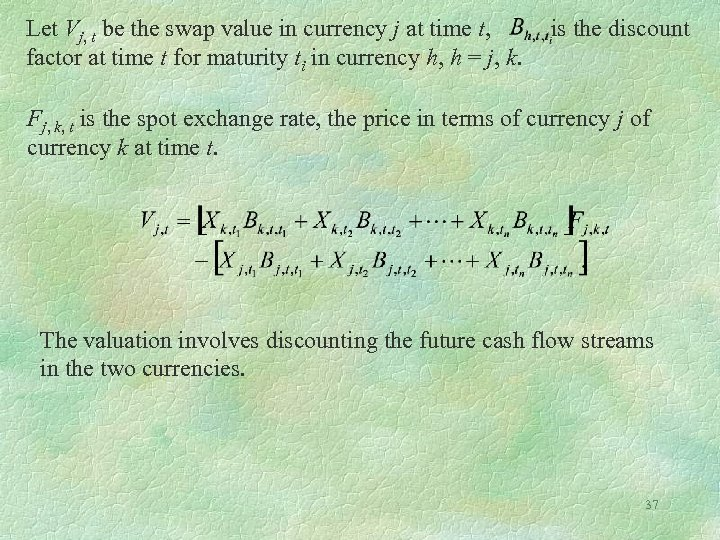Let Vj, t be the swap value in currency j at time t, factor at time t for maturity ti in currency h, h = j, k. is the discount Fj, k, t is the spot exchange rate, the price in terms of currency j of currency k at time t. The valuation involves discounting the future cash flow streams in the two currencies. 37